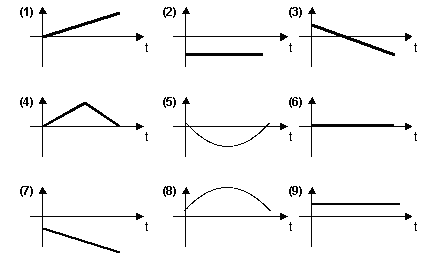Teaching Physics with the Physics Suite Edward F. Redish

Projectile graphs

 A popgun is angled so that it shoots a small dense ball through the air as shown in the figure. a. Sketch the path that the ball will follow on the figure.For the graphs shown at the right, the horizontal axis represents the time. The vertical axis is unspecified. For each of the following quantities, select the letter of the graph that could provide a correct graph of the quantity for the ball in the situation shown (if the vertical axis were assigned the proper units). Use the x and y coordinates shown in the picture. If none of the graphs could work write N. The time graphs begin just after the ball leaves the gun. y coordinate x component of the velocity y component of the net force y component of the velocity x coordinate y component of the acceleration x component of the net forceNot finding what you wanted? Check the Site Map for more information.